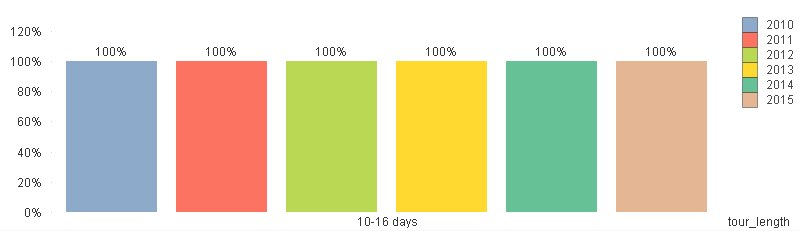New to QlikView

Discussion board where members can get started with QlikView.

Announcements
QlikWorld 2020: Join us May 11 - 14, 2020 in Phoenix, AZ. Register early and save \$400. Learn MoreNot applicable

The data changes when Zoom-In Charts

Hi all, have you noticed this: when you use the mouse and try to zoom in in a chart, the data shown on the chart will change. How to keep the data fixed even when you zoom-in?

Example: this is the original chart.when I zoom-in to "10-16 days": the chart will become:Notice the date all changed to 100%. This is not what we want.

How can I keep the data fixed when I zoom-in and zoom-out?

Thanks!

Tags (2)
1 Solution

Accepted SolutionsMVP & Luminary

Re: The data changes when Zoom-In Charts

It should sit just after the sum statement:

sum({1} if(fiscal = \$(vLastFY-3) and [deposit_date] <=

makedate(year(today())-4, month(date(today())), day(date(today()))), Net_Bookings))

9 Replies
HighlightedPartner

Re: The data changes when Zoom-In Charts

Hi,

how is the expression of the table?

can you post the value of the current selection before and after the zoom?Not applicable

Re: The data changes when Zoom-In Charts

Add in your set analysis of the expression of the cart this filter:

ex.

...sum({<...,tour_lenght={"*"}>Value}...

Valued Contributor II

Re: The data changes when Zoom-In Charts

You'er not 'zooming in' per se, you're filtering the chart, whcih is showing just one set of data. That set is 100% of the new total.MVP & Luminary

Re: The data changes when Zoom-In Charts

I presume your expression is something like:

sum(Value) / sum(TOTAL Value)

Total in this case is the Total of the currently selected values.  You want to ignore selections for this field, so the expression would become:

sum(Value) / sum({1}TOTAL Value)

The {1} is Set Analysis syntax for 'ignore all selections'.  If you want to keep the impact of some selections, but ignore others (eg. only ignore tour_length selections) you would require something like:

sum(Value) / sum({<tour_length=>}TOTAL Value)

If there are multiple fields you need to ignore you need to comma separate them:

sum(Value) / sum({<tour_length=,OtherFeld1=,OtherField2=>}TOTAL Value)

Hopefully that will put you on the right track...

SteveNot applicable

Re: The data changes when Zoom-In Charts

Hi Diego,

The expression:

there are 6 expressions, 1 for each year, the expression looks like:

sum(if([deposit_date] <=

makedate(year(today())-1, month(date(today())), day(date(today())))

and fiscal = vCurrentFY, Net_Bookings)

)/vCurrentFY_NetBooking_YTD_FutureFY

current selection:Not applicable

Re: The data changes when Zoom-In Charts

Hi Steve, this is inspiring,

my expression is

sum(

if(date([deposit_date]) <=

makedate(year(today())-5, month(today()), day(today()))

and fiscal = \$(vLastFY-3), Net_Bookings)

)/\$(vLastFY_3_NetBooking_YTD_FutureFY)

and the vLastFY_3_NetBooking_YTD_FutureFY is dynamic variable :

sum(if(fiscal = \$(vLastFY-3) and [deposit_date] <=

makedate(year(today())-4, month(date(today())), day(date(today()))), Net_Bookings))

How should I use {1} here?MVP & Luminary

Re: The data changes when Zoom-In Charts

Hi Xuan,

It looks like it is the vCurrentFY_NetBooking_YTD_FutureFY variable that needs the Set Analysis code to ignore selections added in.

SteveMVP & Luminary

Re: The data changes when Zoom-In Charts

It should sit just after the sum statement:

sum({1} if(fiscal = \$(vLastFY-3) and [deposit_date] <=

makedate(year(today())-4, month(date(today())), day(date(today()))), Net_Bookings))Not applicable

Re: The data changes when Zoom-In Charts

Thanks Steve, problem solved!